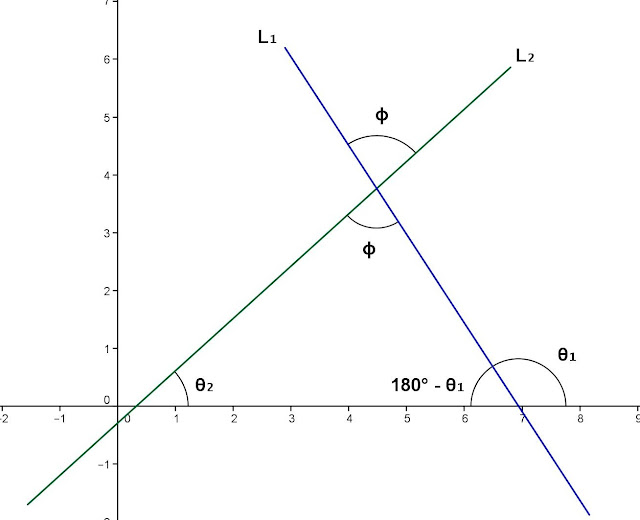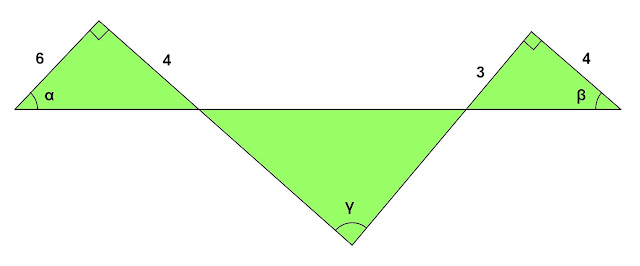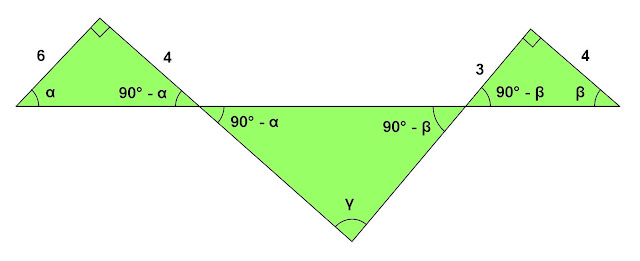## Tuesday, July 30, 2013

### Approximation - Error Problem, 4

Category: Differential Calculus, Solid Geometry

"Published in Newark, California, USA"

Find the approximate formula for the volume of a thin right circular cylindrical shell if r, h, and t denote respectively the radius, length, and thickness.

Solution:

To illustrate the problem, it is better to draw the figure as followsPhoto by Math Principles in Everyday Life

We know that the volume of a right circular cylinder is given by the formula

Take the differential on both sides of the equation, we have

Since the right circular cylinder has a uniform thickness which is t, then it follows that

The above equation becomes

Therefore, the volume of a thin right circular cylindrical shell is

## Monday, July 29, 2013

### Derivation - Angle Between Two Lines

Category: Analytic Geometry, Trigonometry

"Published in Newark, California, USA"

Prove that the formula for getting the angle between two lines is

Solution:

To derive the formula for getting the angle between two lines, let's draw the two intersecting lines in Rectangular Coordinate System as follows:Photo by Math Principles in Everyday Life

L1 is the first line that is inclined to the left and θ1 is the angle of inclination of a line in a counterclockwise direction from x-axis.

L2 is the second line that is inclined to the right and θ2 is the angle of inclination of a line in a counterclockwise direction from x-axis.

As you notice in the figure that L1, L2, and x-axis are the sides of a triangle. Let's label further the figure above as followsPhoto by Math Principles in Everyday Life

The sum of the interior angles of a triangle is calculated as follows

Take tangent on both sides of the equation, we have

Substitute the right side of the equation with the slope of two lines as followsPhoto by Math Principles in Everyday Life

The slope of L1 is

The slope of L2 is

Therefore, the formula for getting the angle between two lines is

## Sunday, July 28, 2013

### Three Triangles - Three Unknown Angles

Category: Trigonometry, Plane Geometry

"Published in Suisun City, California, USA"

Refer to the figure. Show that α + β = γ, and find tan γ.Photo by Math Principles in Everyday Life

Solution:

Referring to the given figure abovePhoto by Math Principles in Everyday Life

Since the two small triangles are right triangles, then we can get the other angle of two triangles as followsPhoto by Math Principles in Everyday Life

The two vertices of a big triangle are vertical angles with the other two triangles. The sum of the interior angles of a big triangle is calculated as follows

Therefore,

Consider again the figure abovePhoto by Math Principles in Everyday Life

We can get the tangent of α and β as follows

If

Take tangent on both sides of the equation, we have

Substitute the values of tan α and tan β to the above equation

Therefore,